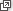# Year 6

## Number and Algebra

### Whole numbers with all four operations

 Mari and family 4 Mari’s mum and dad 2 Mari’s sister 1 Uyen and family 4 Michael and family 4 Cate and partner 2 Janine and family 5 Lauren and family 3 Michael and partner 2 TOTAL 27

When we work out how many people will attend a party, when we calculate the difference between the age of two people, when we find the number of pencils in a box by multiplying the number of packets of pencils in the box by 12 or when we share a packet of chocolate biscuits equally among some friends, we are using addition, subtraction, multiplication and division. We call addition, subtraction, multiplication and division the operations.

Of the four arithmetic operations on numbers, addition is the most natural and, historically, was the first operation developed. Subtraction and addition are inverse operations. For example, 27 − 3 = 24 is equivalent to 27 = 24 + 3. Similarly, multiplication and division are inverse operations. For example, 3 × 4 = 12 is connected to 12 ÷ 3 = 4 and 12 ÷ 4 = 3. The ability to add, subtract, multiply and divide numbers mentally is used in everyday life, such as when we play or watch sport and when we buy a couple of items at the shops.

Formal or written algorithms are useful when larger numbers make mental calculations difficult. While there are many labour-saving devices that will do calculations, a student will not develop a number sense, or fluency, with operations if they move to algorithms and calculators too quickly. There is a range of ways to solve problems using arithmetic and it is important for students to explore these. The commonly taught algorithms have remained in constant use because they are accurate and efficient.

Developing a solid understanding of the operations with whole numbers is essential for understanding later ideas and topics, including arithmetic with fractions and decimals, and with algebra and measurement.

For additional mental strategies see Mental computation.